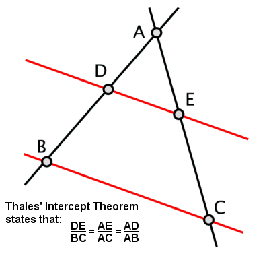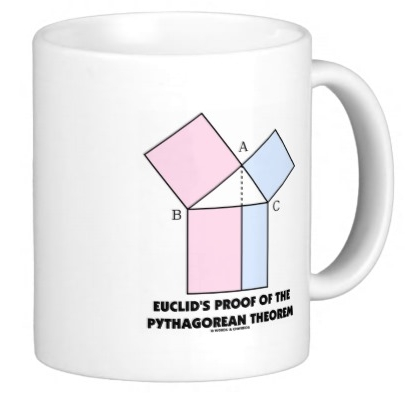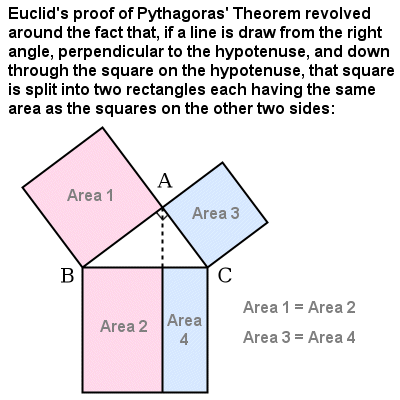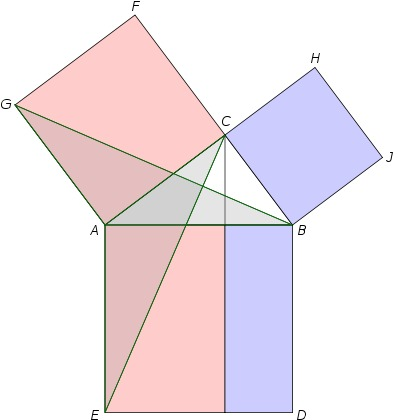a Thales theorem
(circa 500 B.C.)

## A Math Book Inventory

### A List of Books that Elucidate and Popularize Various Topics in Mathematicsanother Thales theorem

! Preliminary ! Under construction !
More book and author information may be added
--- more books may be added ---
and categorizations may be changed.

Go to the Start of the List of Books
(Skip the Introduction)The main idea of Euclids' proof --- pictured on a coffee cupThe main idea of Euclid's proof --- verbally explainedThe triangles that Euclid used to show that
the areas indicated above are indeed equal

(He actually used arguments based on the areas of
parallelograms containing these triangles --- i.e.
the triangles are half of the parallelograms. And
the areas of the parallelograms are the areas of
rectangles of the same height as the parallelogram.)

START of LIST :

Bottom of this
Math Books Inventory page.

OR ...

##### < Go to Start of List, above. >< Go to Top of Page, above. >

Page history

Page was created 2013 Nov 16.

Page was changed 2013 Dec 01.